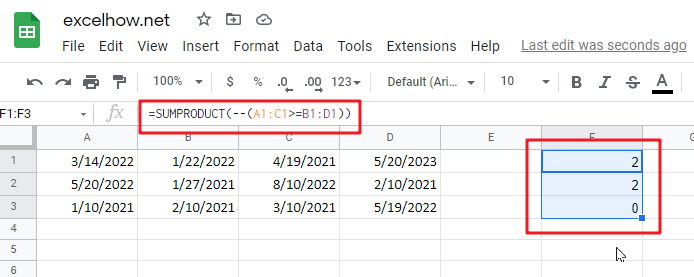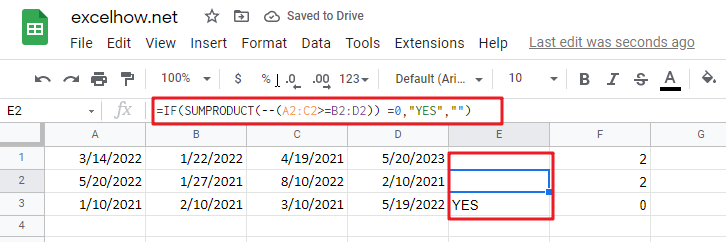# sort dates in chronological order in google sheets

Assume you have a date list that has different date formats, as seen in the accompanying picture. In this instance, google sheets’s Sort function will fail to sort them appropriately. However, you may convert all various date formats to a particular format, such as mm/dd/yyy, and then arrange them chronologically. This post will demonstrate how to sort dates in chronological order and how to check if a range of dates are in chronological order.

## Explanation

This is an excellent illustration of how the SUMPRODUCT function may be used to overcome the inability of the COUNTIF or COUNTIFS functions to operate. In this scenario, the purpose is to verify that all dates within a certain range are in chronological order and to display a “YES” value only when dates are in chronological order.

The argument is pretty straightforward, although maybe not apparent. Rather of ensuring that all dates are bigger than the previous one, we determine if any prior date is greater than the next. If we discover even one, the dates will be out of sequence. They are if we discover none (zero).

At its heart, this formula uses the SUMPRODUCT function to determine the number of dates in A1:C1 that are larger than or equal to B1:D1:

`=SUMPRODUCT(--(A1:C1>=B1:D1))`Due to the fact that we are employing the larger than or equal to (>=) operator, successive duplicate dates will be disallowed.

You can use the following formula based on the IF function, the SUMPRODUCT function, type:

`=IF(SUMPRODUCT(--(A1:C1>=B1:D1)) =0,”YES”,””)`